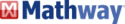0
Thanks

A few words of thanks would be greatly appreciated.

# Solve your Math Problems OnlineIf you need help solving complex mathematical problems, there's a website that can help you out.

Mathway is a online service that enables users to solve mathematical equations from the simplest to the most complex equations. It is free and requires no registration.

## Solve Mathematical Equations with Mathway

Type your equation in the Enter a problem field, choose the type of calculation/operation you'd like to perform from the drop-down list, and then click on the Answer button. Mathway will do the rest:Mathway covers a wide range of topics, including basic math, pre-algebra, algebra, geometry, trigonometry, precalculus, calculus, statistics, finite math, linear algebra, and chemistry.
0
Thanks

A few words of thanks would be greatly appreciated.